# How to Extract Nth Word from Text String Using VBA in Microsoft Excel

In this article, you will learn how to extract the nth word from text string. We will use VBA code to create UDF to find the nth word in a string.

We need to follow the below steps:

• Click on Developer tab
• From Code group, select Visual Basic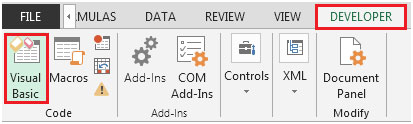• Click on Insert, and then on Module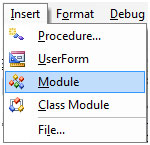• This will create new module.

Enter the following code in the Module

Function ExtractNthWord(x As String, y As Integer)

Dim word() As String

Dim wordCount As Long

word = VBA.Split(x, " ")

wordCount = UBound(word)

If wordCount< 1 Or (y - 1) >wordCount Or y < 0 Then

ExtractNthWord = ""

Else

ExtractNthWord = word(y - 1)

End If

End Function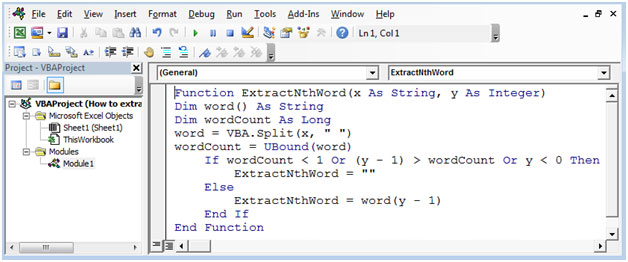• The new UDF formula is created with name ExtractNthWord
•  There are two parameters; first is the lookup cell that needs to be extracted data from & second is the nth number.

Following is the snapshot of the data: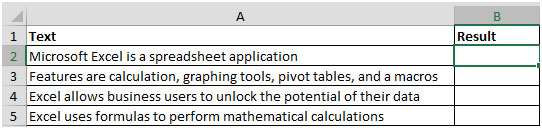• To retrieve 2nd word from column A, the formula will be =ExtractNthWord(A2,2)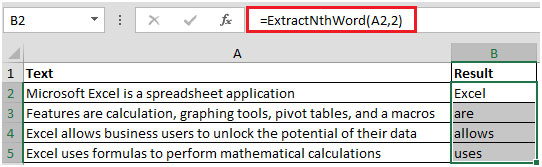• Similarly, to extract 3rd word, the formula will be =ExtractNthWord(A2,3)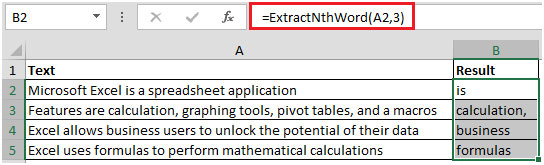• We can use helper cell to store the value of the second argument; this will allow us to see the results faster.
• Here, helper cell is D1 wherein we can enter the nth number of word that we want to see as output.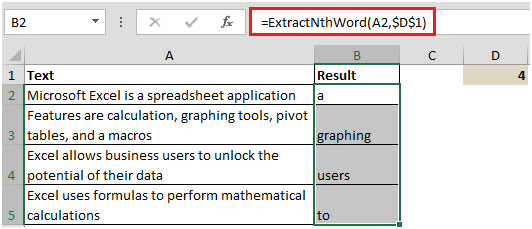Now, we only need to change the number in cell D1 & the results will automatically be changed accordingly.

Terms and Conditions of use

The applications/code on this site are distributed as is and without warranties or liability. In no event shall the owner of the copyrights, or the authors of the applications/code be liable for any loss of profit, any problems or any damage resulting from the use or evaluation of the applications/code.